## Excel Spill Formula Examples

Written by Debra Dalgleish from Contextures Blog

Spill functions can fill neighbouring cells with their results, to create dynamic ranges. Thank you to Dermot Balson, who sent me his Excel workbook with examples for all the new functions (dynamic arrays) in Excel for Office 365. The examples show how VBA functions can spill too, and even some old Excel functions will spill automatically.

### Old Functions Spill

Before we look at a new function, here’s what can happen with an old function, in Excel 365.

This OFFSET formula is entered in cell H19, and it returns 3 rows and 3 columns of data, by spilling into the adjacent rows and columns:

=OFFSET(C4,I17,0,3,3)

The formula refers to cell I17, and you can change the number there, to set the number of rows to offset.### Range Reference Spills

And you don’t even need a function – a simple range reference will spill into adjacent rows and columns. For example, this formula is in cell C19:

• =C6:E8

The formula results spill into the adjacent rows and columns, as far as needed, to show all 9 cells that the formula refers to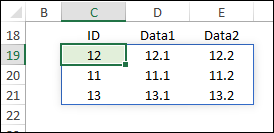### Spill Cells

Here are a few key things to know about the spill formula results:

• If you select any cell in the formula results, a thin blue border appears around the spill range
• The formula can only be edited in the cell where it was entered
• If any other spill cell is selected, the formula can be seen in the Formula bar, in grey font, but it cannot be edited

### UNIQUE Function

UNIQUE is one of the new Excel functions. It lets you pull unique items from another list. The unique items can be from a single column, or you can list unique combinations, from two or more columns.

In the first example in this screenshot, this formula returns a single column of unique IDs from column C.

• =UNIQUE(C5:C11)

The second example returns unique combinations of ID and Data1 values, from columns C and D:

• =UNIQUE(C5:D11)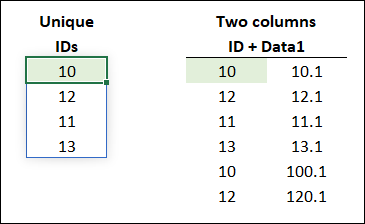### SORT Function

Another new function is SORT, which returns a sorted list. You can return a list that’s sorted by a specific column. Or, you can  sort by two or more columns.

In this example, the list is sorted the first column, in ascending order (1), and then by the second column, in descending order (-1)

• =SORT(C5:E11,{1,2},{1,-1})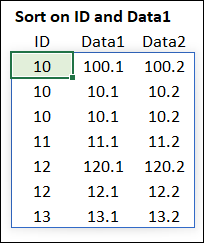### SORTBY Function

The SORTBY function is similar to SORT, but you don’t need the “sort” column in the formula results.

In this screenshot, only the Data2 values (from column E) are in the formula results. The SORTBY function sorted the sample data by Data1 (column C, ascending ) and then by ID (column D, descending).

=SORTBY(E5:E11,C5:C11,-1,D5:D11,-1)### More New Functions

There are a few more new functions – FILTER, SEQUENCE and RANDARRAY. You can see examples of those on the Excel Spill Functions page on my Contextures site.

Here’s a peek at what the SEQUENCE function can do though, in combination with the old TODAY function. This formula creates a 4-week calendar, starting with the current date:

=SEQUENCE(4,7,TODAY())

Above the calendar, the old TEXT function creates the heading row, showing the 3-letter weekday names.

• =TEXT(J102:P102,”DDD”)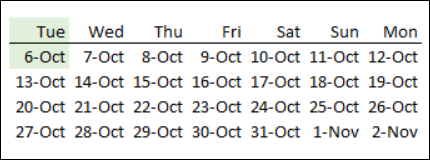Custom VBA Functions

In his Spill Functions workbook, Dermot also shows how custom VBA functions can spill into neighbouring cells.

For example, this simple custom VBA function, SimpleArray, creates a little table and returns on the worksheet.

Here is the function being used on the worksheet, to create an array of 3 rows and 5 columns

• =simplearray(3,5)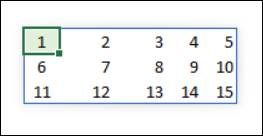And here is the code for the SimpleArray custom VBA function:

```Function SimpleArray(x, y)
Dim i, j, n
ReDim A(x, y)
For i = 1 To x
For j = 1 To y
n = n + 1
A(i, j) = n
Next j
Next i
SimpleArray = A
End Function```

#### More VBA Custom Functions

In the sample file, there are several more VBA custom functions that you can test.

• Simple Array
• Split String into Cells
• Ranking, with tie breaker
• SQL Query
• Compare 2 Lists
• Crosscheck 2 Lists
• Join cells or Lists
• Regular Expression
• Formula Counter

### Get the Spill Formula Workbook

To see all of Dermot’s spill formula examples, and to get the sample file, go to the Excel Spill Functions page on my Contextures site.

The zipped workbook is in xlsx format, and contains macros.

________________________

### Excel Spill Formula Examples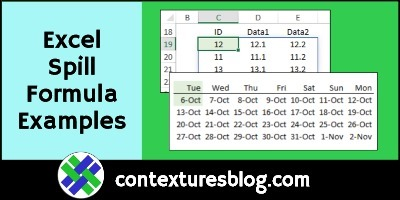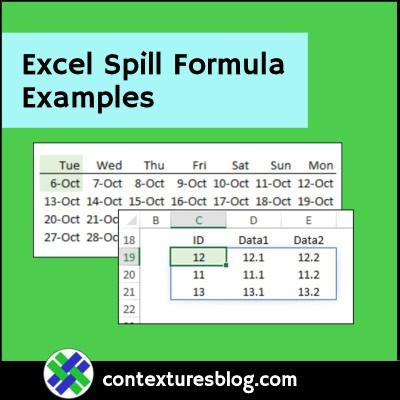________________________

Excel Spill Formula Examples is a post from Contextures Blog and is not allowed to be copied to other sites